## How to Calculate and Solve for Magnetic Flux | Magnetic Field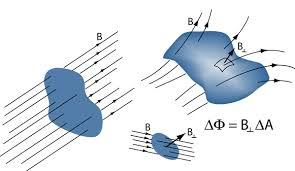The image above represents magnetic flux.

To compute for magnetic flux, two essential parameters are needed and these parameters are Area (A) and Total Magnetic Field (B).

The formula for calculating magnetic flux:

φ = B.A

Where:

φ = Magnetic Flux
A = Area
B = Total Magnetic Field

Let’s solve an example;
Find the magnetic flux with a given area of 32 and a total magnetic field of 18.

This implies that;

A = Area = 32
B = Total Magnetic Field = 18

φ = B.A
φ = (18)(32)
φ = 576

Therefore, the magnetic flux is 576 Wb.

Calculating the Area when the Magnetic Flux and the Total Magnetic Field is Given.

A = φ / B

Where;

A = Area
φ = Magnetic Flux
B = Total Magnetic Field

Let’s solve an example;
Find the area when the magnetic flux is 40 and the total magnetic field is 20.

This implies that;

φ = Magnetic Flux = 40
B = Total Magnetic Field = 20

A = φ / B
A = 40 / 20
A = 2

Therefore, the area is 2.

## How to Calculate and Solve for Viscosity | Ceramics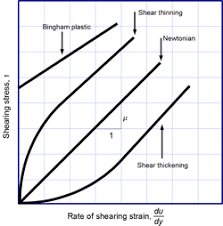The image above represents viscosity.

To compute for viscosity, three essential parameters are needed and these parameters are Force applied (F), Area (A) and Derivation Ratio of Velocity to Distance of Fluid Flow (dv/dy).

The formula for calculating viscosity:

η = F/A / dv/dy

Where:

η = Viscosity
F = Force Applied
A = Area
dv/dy = Derivation Ratio of Velocity to Distance of Fluid Flow

Let’s solve an example;
Find the viscosity when the force applied is 21, area is 14 and derivation ratio of velocity to distance of fluid flow is 19.

This implies that;

F = Force Applied = 21
A = Area = 14
dv/dy = Derivation Ratio of Velocity to Distance of Fluid Flow = 19

η = F/A / dv/dy
η = (21/14) / (19)
η = (1.5) / (19)
η = 0.0789

Therefore, the viscosity is 0.0789 Pa s.

Calculating Force Applied when the Viscosity, the Area and the Derivation ratio of velocity to distance of fluid flow is Given.

F = (η x dv/dy) A

Where;

F = Force Applied
η = Viscosity
A = Area
dv/dy = Derivation Ratio of Velocity to Distance of Fluid Flow

Let’s solve an example;
Find the force applied when the viscosity is 20, the area is 30 and the derivation is 8.

This implies that;

η = Viscosity = 20
A = Area = 30
dv/dy = Derivation Ratio of Velocity to Distance of Fluid Flow = 8

F = (η x dv/dy) A
F = (20 x 8) 30
F = (160) 30
F = 4800

Therefore, the force applied is 4800.

## How to Calculate and Solve for Discharge | Chezy’s Equation | Water Budget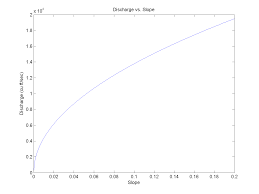The image above represents discharge | Chezy’s Equation.

To compute for discharge, four essential parameters are needed and these parameters are Area (A), Chezy’s Constant (C), Hydraulic Radius (R) and Slope (S).

The formula for calculating discharge:

Q = AC√(RS)

Where;

Q = Discharge
A = Area
C = Chezy’s Constant
S = Slope

Let’s solve an example;
Find the discharge when the area is 25, the chezy’s constant is 16, the hydraulic radius is 9 and the slope is 21.

This implies that;

A = Area = 25
C = Chezy’s Constant = 16
R = Hydraulic Radius = 9
S = Slope = 21

Q = AC√(RS)
Q = (25)(16)√((9)(21))
Q = (25)(16)√(189)
Q = (25)(16)(13.74)
Q = 5499.09

Therefore, the discharge according to Chezy’s equation is 5499.09.

Calculating the Area when the Discharge, the Chezy’s Constant, the Hydraulic Radius and the Slope is Given.

A = Q / C √RS

Where;

A = Area
Q = Discharge
C = Chezy’s Constant
S = Slope

Let’s solve an example;
Find the area when the discharge is 40, the chezy’s constant is 10, the hydraulic radius is 5 and the slope is 9.

This implies that;

Q = Discharge = 40
C = Chezy’s Constant = 10
R = Hydraulic Radius = 5
S = Slope = 9

A = Q / C √RS
A = 40 / 10 √(5)(9)
A = 40 / 10 √45
A = 40 / 10 (6.708)
A = 40 / 67.08
A = 0.596

Therefore, the area is 0.596.

## How to Calculate and Solve for Discharge | Slope Area Method | Water Budget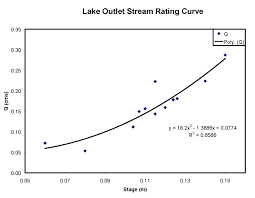The image above represents discharge | slope area method.

To compute for discharge | slope area method, four essential parameters are needed and these parameters are Manning’s Co-efficient (N), Area (A), Hydraulic Radius (R) and Slope (S).

The formula for calculating discharge | slope area method:

Q = (A / N) R2/3 S1/2

Where;

Q = Discharge
N = Manning’s Co-efficient
A = Area
S = Slope

Let’s solve an example;
Find the discharge when the manning’s co-efficient is 4, the area is 30, the hydraulic radius is 15 and the slope is 22.

This implies that;

N = Manning’s Co-efficient = 4
A = Area = 30
R = Hydraulic Radius = 15
S = Slope = 22

Q = (A / N) R2/3 S1/2
Q = (30 / 4) (15)2/3 (22)1/2
Q = (7.5) (6.08) (4.69)
Q = 213.96

Therefore, the discharge is 213.96.

## How to calculate and Solve for Shear Stress | Rock Mechanics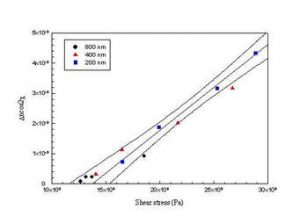The image above represents shear stress.

To compute for shear stress, two essential parameters are needed and these parameters are Shear Force (ΔS) and Area (ΔA).

The formula for calculating the shear stress:

τ = ΔS / ΔA

Where:

τ = Shear Stress
ΔS = Shear Force
ΔA = Area

Let’s solve an example;
Find the shear stress when the shear force is 16 with an area of 30.

This implies that;

ΔS = Shear Force = 16
ΔA = Area = 30

τ = ΔS / ΔA
τ = 16 / 30
τ = 0.53

Therefore, the shear stress is 0.53 Pa.

Calculating the Shear Force when the Shear Stress and Area is Given.

ΔS = τ x ΔA

Where;

ΔS = Shear Force
τ = Shear Stress
ΔA = Area

Let’s solve an example;
Find the shear force with a shear stress of 40 and an area of 12.

This implies that;

τ = Shear Stress = 40
ΔA = Area = 12

ΔS = τ x ΔA
ΔS = 40  x 12
ΔS = 480

Therefore, the Shear Force is 480.

## How to Calculate and Solve for Normal Stress | Rock Mechanics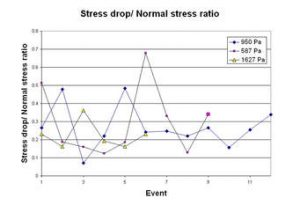The image above represents normal stress.

To calculate for normal stress, two essential parameters are needed and these parameters are normal force (ΔN) and area (ΔA).

The formula for calculating the normal stress:

σn = ΔN / ΔA

Where;

σn = Normal Stress
ΔN = Normal Force
ΔA = Area

Let’s solve an example;
Calculate the normal stress with a normal force of 12 and an area of 22.

This implies that;

ΔN = Normal Force = 12
ΔA = Area = 22

σn = ΔN / ΔA
σn = 12 / 22
σn = 0.54

Therefore, the normal stress is 0.54 Pa.

Calculating the Normal Force when the Normal Stress and Area is Given.

ΔN = σn x ΔA

Where;

ΔN = Normal Force
σn = Normal Stress
ΔA = Area

Let’s solve an example;
Calculate the normal force when the normal stress is 25 with an area of 10.

This implies that;

σn = Normal Stress = 25
ΔA = Area = 10

ΔN = σn x ΔA
ΔN = 25 x 10
ΔN = 250

Therefore, the normal force is 250.

## How to Calculate and Solve for Infinite Acting Period | The Calculator Encyclopedia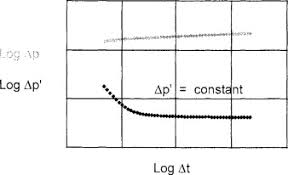The image represents the infinite acting period.

To compute the infinite acting period, six essential parameters are needed and these parameters are permeability (k), porosity (φ), well drainage area (A), viscosity (μ), Dimensionless Time to End of Infinite Acting Period ((tDA)eia) and total compressibility (CT).

The formula for calculating the infinite acting period:

teia = [φ μ CT A / 0.000263K] (tDA)eia

Where;

teia = Infinite Acting Period
K = Permeability
φ = Porosity
A = Well Drainage Area
μ = Viscosity
(tDA)eia = Dimensionless Time to End of Infinite Acting Period
CT = Total Compressibility

Let’s solve an example;
Given that the permeability is 21, porosity is 15, well drainage area is 32, viscosity is 26, dimensionless time to end of infinite acting period is 44 and total compressibility is 34.
Find the infinite acting period?

This implies that;

K = Permeability = 21
φ = Porosity = 15
A = Well Drainage Area = 32
μ = Viscosity = 26
(tDA)eia = Dimensionless Time to End of Infinite Acting Period = 44
CT = Total Compressibility = 34

teia = [φ μ CT A / 0.000263K] (tDA)eia
teia = [15 x 26 x 34 x 32 / 0.000263 x 21] 44
teia = [424320 / 0.005523] 44
teia = [76827810.972] 44
teia = 3380423682.78

Therefore, the infinite acting period is 3380423682.78 s.

## How to Calculate and Solve for True Stress | The Calculator Encyclopedia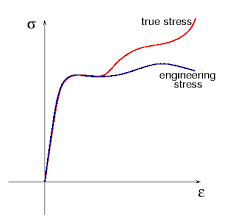The image above represents the true stress.

To compute for the true stress, two essential parameters are needed and these parameters are force (F) and instantaneous area (Ai).

The formula for calculating true stress:

σT = F / Ai

Where;
T = True Stress
F = Force
Ai = Instantaneous Area

Let’s solve an example;
Find the true stress when the instantaneous area is 60 with a force of 25.

This implies that;
F = Force = 25
Ai = Instantaneous Area = 60

σT = F / Ai
σT = 25 / 60
σT = 0.416

Therefore, the true stress is 0.416 Pa.

Calculating the Force when True Stress and Instantaneous Area is Given.

F = Ai x σT

Where;
F = Force
σT = True Stress
Ai = Instantaneous Area

Let’s solve an example;
Find the force when the instantaneous area is 30 with a true stress of 15.

This implies that;
σT = True Stress = 15
Ai = Instantaneous Area = 30

F = Ai x σT
F = 30 x 15
F = 450

Therefore, the force is 450.

## How to Calculate and Solve for the Height, Base Edge and Area of a Square Pyramid | Nickzom Calculator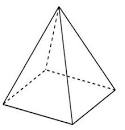The image above is a square pyramid.

To compute the area of a square pyramid, two essential parameters are needed and this parameters are base edge (a) and height (h).

The formula for calculating the area of a square pyramid:

A = a² + 2a√(( / 4) + h²)

Where;
A = Area of a square pyramid
a = Base edge of the square pyramid
h = Height of the square pyramid

Let’s solve for an example;
Find the area of a square pyramid when the base edge (a) given is 8 cm with a height (h) of 12 cm.

This implies that;
a = Base edge of the square pyramid = 8 cm
h = Height of the square pyramid = 12 cm

A = a² + 2a√(( / 4) + h²)
A = 8² + 2 x 8√(( / 4) + 12²)
A = 64 + 16√((64 / 4) + 144)
A = 64 + 16√(16 + 144)
A = 64 + (16)(√160)
A = 64 + (16)(12.65)
A = 64 + 202.3857
A = 266.386

Therefore, the area of the square pyramid is 266.386 cm2.

## How to Calculate and Solve for the Area of an Ellipsoid | The Calculator Encyclopedia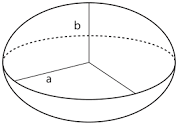The image above is an ellipsoid.

To compute the area of an ellipsoid, three essential parameters are needed and this parameters are axis (a)axis (b) and axis (c).

The formula for calculating the area of an ellipsoid:

A = 4π((ab)1.6 + (ac)1.6 + (bc)1.63)1 / 1.6

Where;
A = Area of the ellipsoid
a = Axis of the ellipsoid
b = Axis of the ellipsoid
c = Axis of the ellipsoid

Let’s solve an example;
Find the the area of an ellipsoid when the axis (a) of the ellipsoid is 12 cm, axis (b) of the ellipsoid is 6 cm and axis (c) of the ellipsoid is 2 cm.

This implies that;
a = Axis of the ellipsoid = 12 cm
b = Axis of the ellipsoid = 6 cm
c = Axis of the ellipsoid = 2 cm

A = 4π((ab)1.6 + (ac)1.6 + (bc)1.63)1 / 1.6
A = 4π(((12)(6))1.6 + ((12)(2))1.6 + ((6)(2))1.63)1 / 1.6
A = 4π(((72)1.6 + (24)1.6 + (12)1.6)3)1 / 1.6
A = 4π(((936.98) + (161.56) + (53.29))3)1 / 1.6
A = 4π((1151.84)3)1 / 1.6
A = 4π(383.946)1 / 1.6
A = 4π(383.946)0.625
A = 4π(41.2258)
A = (12.566)(41.2258)
A = 518.059

Therefore, the area of the ellipsoid is 518.059 cm2.